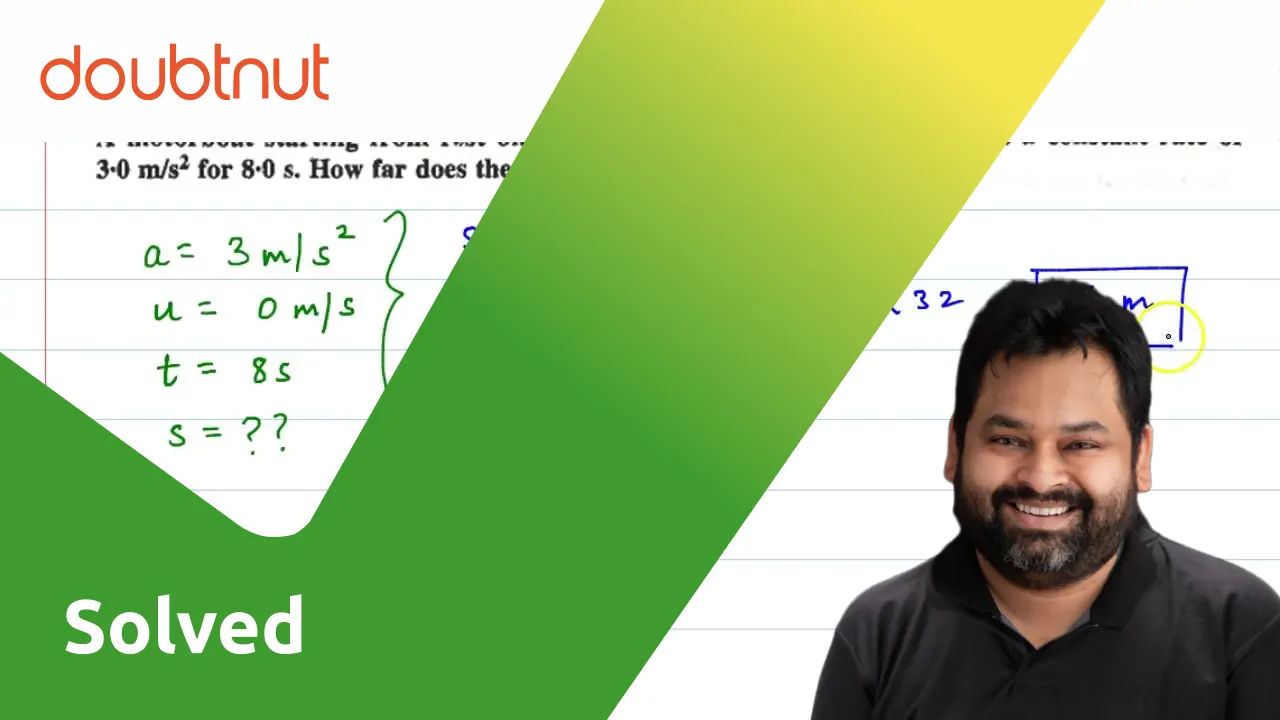Doubtnut App - Get 50 Lakh+ video solutions better than GoogleInstall Now

# A motorboat starting from rest on a lake acceleration line at a constant rate of 3.0m/s2 for 8.0s. How far does the boat travel during this time ?

Text Solution
Verified by Experts

## Here, initial velocity, u=0, acceleration, a=3.0m/s2 time, t=8.0s, distance travelled, s=? From s=ut+12at2, s=0×8+12×3.0(8.0)2=96m